## Determinants of Price to Book Ratios

The price-book value ratio can be related to the same fundamentals that determine value in discounted cashflow models. Since this is an equity multiple, we will use an equity discounted cash flow model – the dividend discount model – to explore the determinants. The value of equity in a stable growth dividend discount model can be written as:where,

P0 = Value of equity per share today

DPS1 = Expected dividends per share next year

ke = Cost of equity

gn = Growth rate in dividends (forever)

Substituting in for DPS1 = (EPS1)(Payout ratio), the value of the equity can be written as:Defining the return on equity (ROE)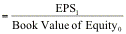, the value of equity can be written as: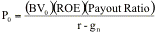Rewriting in terms of the PBV ratio,If we define return on equity using contemporaneous earnings, ROE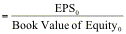, the price to book ratio can be written as: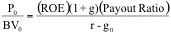The PBV ratio is an increasing function of the return on equity, the payout ratio and the growth rate and a decreasing function of the riskiness of the firm.

This formulation can be simplified even further by relating growth to the return on equity.

g = (1 - Payout ratio) * ROE

Substituting back into the P/BV equation,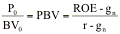The price-book value ratio of a stable firm is determined by the differential between the return on equity and its cost of equity. If the return on equity exceeds the cost of equity, the price will exceed the book value of equity; if the return on equity is lower than the cost of equity, the price will be lower than the book value of equity. The advantage of this formulation is that it can be used to estimate price-book value ratios for private firms that do not pay out dividends.

###### Illustration 19.1: Estimating the PBV ratio for a stable firm - Volvo

Volvo had earnings per share of 11.04 Swedish Kroner (SEK) in 2000 and paid out a dividend of 7 SEK per share, which represented 63.41% of its earnings. The growth rate in earnings and dividends, in the long term, is expected to be 5%. The return on equity at Volvo is expected to be 13.66%. The beta for Volvo is 0.80 and the riskfree rate in Swedish Kroner is 6.1%.

Current Dividend Payout Ratio = 63.41%

Expected Growth Rate in Earnings and Dividends = 5%

Return on Equity = 13.66%

Cost of Equity = 6.1% + 0.80*4% = 9.30%

PBV Ratio based on fundamentalsSince the expected growth rate in this case is consistent with that estimated by fundamentals, the price to book ratio could also have been estimated from the return differences.

Fundamental growth rate = (1 – payout ratio)(ROE) = (1-0.6341)(0.1366) = .05 or 5%

PBV ratio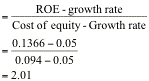Volvo was selling at a P/BV ratio of 1.10 on the day of this analysis (May 2001), making it significantly under valued. The alternative interpretation is that the market is anticipating a much lower return on equity in the future and pricing Volvo based upon this expectation.

###### Illustration 19.2: Estimating the price-book value ratio for a 'privatization' candidate - Jenapharm (Germany)

One of the by-products of German reunification was the Treuhandanstalt, the German privatization agency set up to sell hundreds of East German firms to other German companies, individual investors and the public. One of the handful of firms that seemed to be a viable candidate for privatization was Jenapharm, the most respected pharmaceutical manufacturer in East Germany. Jenapharm, which was expected to have revenues of 230 million DM in 1991, also was expected to report net income of 9 million DM in that year. The firm had a book value of assets of 110 million DM and a book value of equity of 58 million DM at the end of 1990.

The firm was expected to maintain sales in its niche product, a contraceptive pill, and grow at 5% a year in the long term, primarily by expanding into the generic drug market. The average beta of pharmaceutical firms traded on the Frankfurt Stock exchange was 1.05, though many of these firms had much more diversified product portfolios and less volatile cashflows. Allowing for the higher leverage and risk in Jenapharm, a beta of 1.25 was used for Jenapharm. The ten-year bond rate in Germany at the time of this valuation in early 1991 was 7% and the risk premium for stocks over bonds is assumed to be 3.5%.

Expected Net Income = 9 mil DM

Return on EquityCoat of Equity = 7% + 1.25 (3.5%) = 11.375%

Price/Book Value Ratio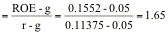Estimated MV of equity### PBV Ratio for a high growth firm

The price-book value ratio for a high growth firm can also be related to fundamentals. In the special case of the two-stage dividend discount model, this relationship can be made explicit simply. The value of equity of a high growth firm in the two-stage dividend discount model can be written as:

Value of Equity = Present Value of expected dividends + Present value of terminal price

When the growth rate is assumed to be constant after the initial high growth phase, the dividend discount model can be written as follows: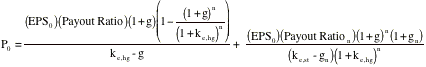where,

g = Growth rate in the first n years

Payout = Payout ratio in the first n years

gn = Growth rate after n years forever (Stable growth rate)

Payoutn = Payout ratio after n years for the stable firm

ke = Cost of equity (hg: high growth period; st: stable growth period)

Rewriting EPS0 in terms of the return on equity, EPS0 = (BV0)(ROE), and bringing BV0 to the left hand side of the equation, we get: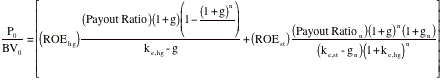where ROE is the return on equity and ke is the cost of equity.

The left hand side of the equation is the price book value ratio. It is determined by:

(a) Return on equity: The price-book value ratio is an increasing function of the return on equity.

(b) Payout ratio during the high growth period and in the stable period: The PBV ratio increases as the payout ratio increases, for any given growth rate.

(c) Riskiness (through the discount rate r): The PBV ratio becomes lower as riskiness increases; the increased risk increases the cost of equity.

(d) Growth rate in Earnings, in both the high growth and stable phases: The PBV increases as the growth rate increases, in either period, holding the payout ratio constant.

This formula is general enough to be applied to any firm, even one that is not paying dividends right now. Note, in addition, that the fundamentals that determine the price to book ratio for a high growth firm are the same as the ones for a stable growth firm – the payout ratio, the return on equity, the expected growth rate and the cost of equity.

In Chapter 14, we noted that firms may not always pay out what they can afford to and recommended that the free cashflows to equity be substituted in for the dividends in those cases. You can, in fact, modify the equation above to state the price to book ratio in terms of free cashflows to equity.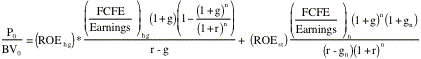The only substitution that we have made is the replacement of the payout ratio by the FCFE as a percent of earnings.

###### Illustration 19.3: Estimating the PBV ratio for a high growth firm in the two-stage model

Assume that you have been asked to estimate the PBV ratio for a firm that is expected to be in high growth for the next five years. The firm has the following characteristics:

EPS Growth rate in first five years = 20%        Payout ratio in first five years = 20%

EPS Growth rate after five years = 8%             Payout ratio after five years = 68%

Beta = 1.0                                                          Riskfree rate = T.Bond Rate = 6%

Return on equity = 25%

Cost of equity = 6% + 1(5.5%)= 11.5%The estimated PBV ratio for this firm is 7.89.

###### Illustration 19.4: Estimating the Price/Book Value Ratio for a high growth firm using FCFE - Nestle

In Chapter 14, we valued Nestle using a two-stage FCFE model. We summarize the inputs we used for that valuation in the Table 19.1.

Table 19.1: Nestle – Summary of Inputs

 High Growth Stable Growth Length 10 years Forever after year 10 ROE 22.98% 15% FCFE/Earnings 68.35% 73.33% Growth rate 7.27% 4% Cost of Equity 8.47% 8.47%

The price-book value ratio, based upon these inputs, is calculated below: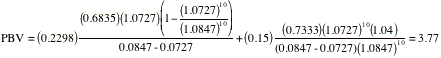Nestle traded at a price-book value ratio of 4.40 in May 2001, which would make it over valued.

Again, in this valuation, we have preserved consistency by setting the growth rate equal to the product of the return on equity and the equity reinvestment rate (1- FCFE/ Earnings).

Growth rate during high growth         = ROE (1- FCFE/Earnings)

= 1.2298 (1 - 0.6835) = 0.0727

Growth rate during stable growth = ROE (1- FCFE/Earnings) = 0.15 (1-0.7333) = 0.04

### PBV Ratios and Return on Equity

The ratio of price to book value is strongly influenced by the return on equity. A lower return on equity affects the price-book value ratio directly through the formulation specified in the prior section and indirectly by lowering the expected growth or payout.

Expected growth rate = Retention Ratio * Return on Equity

The effects of lower return on equity on the price-book value ratio can be seen by going back to Illustration 19.3 and changing the return on equity for the firm that we valued in that example.

###### Illustration 19.5:  Return on Equity and Price-Book Value

In Illustration 19.3, we estimated a price to book ratio for the firm of 7.89, based upon a return on equity of 25%. This return on equity, in turn, allowed the firm to generate growth rates of 20% in high growth and 8% in stable growth.

Growth rate in first five yearsGrowth rate after year 5If the firm's return on equity drops to 12%, the price/book value will reflect the drop. The lower return on equity will also lower expected growth in the initial high growth period:

Expected growth rate (first five years)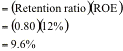After year 5, either the retention ratio has to increase or the expected growth rate has to be lower than 8%. If the retention ratio is adjusted,

New retention ratio after year 5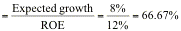New payout ratio after year 5 = 1 - Retention ratio = 33.33%

The new price-book value ratio can then be calculated as follows: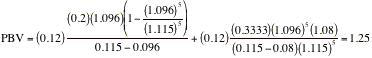The drop in the ROE has a two-layered impact. First, it lowers the growth rate in earnings and/or the expected payout ratio, thus having an indirect effect on the P/BV ratio. Second, it reduces the P/BV ratio directly.

The price-book value ratio is also influenced by the cost of equity, with higher costs of equity leading to lower price-book value ratios. The influence of the return on equity and the cost of equity can be consolidated in one measure by taking the difference between the two – a measure of excess equity return. The larger the return on equity relative to the cost of equity, the greater is the price-book value ratio. In the illustration above for instance, the firm, which had a cost of equity of 11.5%, went from having a return on equity that was 13.5% greater than the required rate of return to a return on equity that barely broke even (0.5% greater than the required rate of return). Consequently, its price-book value ratio declined from 7.89 to 1.25. The following graph shows the price-book value ratio as a function of the difference between the return on equity and required rate of return.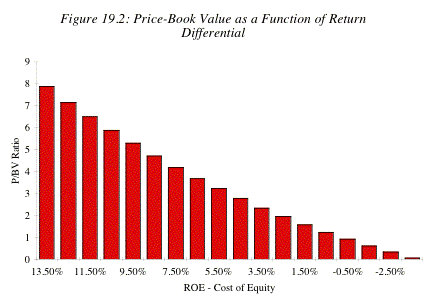Note that when the return on equity is equal to the cost of equity, the price is equal to the book value.

### The Determinants of Return on Equity

The difference between return on equity and the required rate of return is a measure of a firm's capacity to earn excess returns in the business in which it operates. Corporate strategists have examined the determinants of the size and expected duration of these excess profits (and high ROE) using a variety of frameworks. One of the better known is the "five forces of competition" framework developed by Porter. In his approach, competition arises not only from established producers producing the same product but also from suppliers of substitutes and from potential new entrants into the market. Figure 19.3 summarizes the five forces of competition:

Figure 19.3: Forces of Competition and Return on Equity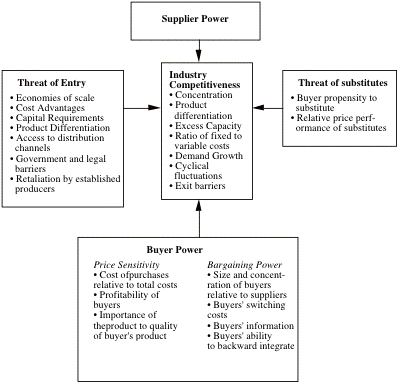In Porter's framework, a firm is able to maintain a high return on equity because there are significant barriers to entry by new firms or because the firm has significant advantages over its competition. The analysis of the return on equity of a firm can be made richer and much more informative by examining the competitive environment in which it operates. There may also be clues in this analysis to the future direction of the return on equity.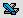eqmult.xls: This spreadsheet allows you to estimate the price earnings ratio for a stable growth or high growth firm, given its fundamentals.